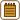## The van der Waals Equation

Nitrogen at an atmospheric pressure of 101 325 Pa and a temperature of 273.15 K undergoes isothermal compression to one tenth of its initial volume. Mass of the nitrogen is 28 g. Determine the work performed by the gas, considering that its behaviour is defined by the van der Waals equation.

• #### Hint: Work of the Nitrogen

Think about how to calculate the work done by the nitrogen if its pressure is not constant.

• #### Hint: Expressing the Pressure

To express pressure p as a function of volume V, we should use the van der Waals equation.

Think about which quantities in the equation are known and which ones we still have to express.

• #### Notation

 T = 273.15 K temperature at which the process takes place p1 = 101 325 Pa atmospheric pressure m = 28 g = 0.028 kg mass of the nitrogen V2 = 0.1V1 volume of the nitrogen after compression ΔW = ? work of the gas

From the Tables:

 Mm = 28 g mol−1 = 0.028 kg mol−1 molar mass of nitrogen R = 8.31 Jmol−1K−1 molar gas constant a = 0.137 Jm3mol−2 “van der Waals” constant for nitrogen b = 38.7·10−6 m3mol−1 “van der Waals” constant for nitrogen
• #### Analysis

To calculate the work done by the nitrogen, we have to use integration because the pressure is not constant (it is a function of volume).

We express the nitrogen pressure from the van der Waals equation and integrate the obtained function with respect to the volume. Limits of the integral are initial and final volume of the nitrogen.

Amount of nitrogen is determined by its total mass and molar mass of nitrogen.

To calculate the initial volume of nitrogen, the ideal gas law is to be used. Since the gas was at the beginning of the process under atmospheric pressure and only later it has been compressed and hence its pressure increased, such simplifying assumption is fairly justified (the ideal gas law applies to the gas at low pressure).

• #### Solution

Work performed at constant pressure is determined by the formula W = p(V2 − V1).

However, in our case pressure p is not constant (the process is not isobaric), which means that we will have to use a more general formula:

$W = \int\limits_{V_1}^{V_2}p(V)\, \text{d}V,$

where V1 is the initial volume of nitrogen and V2 its volume after the compression.

To use this formula, we need to express pressure p as a function of volume V. To do so, we use the van der Waals equation

$\left(p+\frac{n^{2}a}{V^{2}}\right)(v-nb)=nRT,$

where p is pressure, V volume, T thermodynamic temperature, R the gas constant, n amount of substance, and finally a and b are the constants that describe non-ideality of the gas.

Now we adjust the van der Waals equation so that pressure is explicitly expressed through other variables. It holds true:

$p+\frac{n^{2}a}{V^{2}}=\frac{nRT}{V-nb},$

and thus,

$p=\frac{nRT}{V-nb}-\frac{n^{2}a}{V^{2}}.$

Then we substitute the just obtained formula for pressure in the original formula for the performed work W, and we can proceed with integration:

$W=\int_{V_{1}}^{V_{2}}{p}\,\mathrm{d}V=\int_{V_{1}}^{0.1V_{1}}\left({\frac{nRT}{V-nb}-\frac{n^{2}a}{V^{2}}}\right)\mathrm{d}V=$

we split the integral into two simpler ones and factor out the constants

$=nRT\int_{V_{1}}^{0.1V_{1}}{\frac{\mathrm{d}V}{V-nb}}-n^{2}a\int_{V_{1}}^{0.1V_{1}}{\frac{\mathrm{d}V}{V^{2}}}=$

now integrate

$=nRT\left[\ln(V-nb)\right]_{V_{1}}^{0.1V_{1}}+n^{2}a\left[\frac{1}{V}\right]_{V_{1}}^{0.1V_{1}}=$

and substitute the limits

$=nRT\ln{\left(\frac{0.1V_{1}-nb}{V_{1}-nb}\right)}+n^{2}a\left(\frac{1}{0.1V_{1}}-\frac{1}{V_{1}}\right).$

After adjusting the expression in the second pair of brackets, we get the following relation for the performed work

$W=nRT\ln{\left(\frac{0.1V_{1}-nb}{V_{1}-nb}\right)}+\frac{9n^{2}a}{V_{1}}.$

In order to calculate the work, we also need to determine the amount of nitrogen n and initial volume V1.

Amount of substance can be easily determined by the following formula

$n=\frac{m}{M_{m}},$

where m is the total mass of nitrogen and Mm is its molar mass.

Initial volume of nitrogen V1 could be calculated directly from the van der Waals equation. However, we would have had to solve a cubic equation (i.e. unknown volume is raised to the third power). Unfortunately, such an equation is not easy to calculate; usually, it is necessary to use some more complex numerical method. Therefore, we will simplify the task and use the ideal gas law to calculate the original volume

$p_1V_{1}=\frac{m}{M_{m}}RT,$

from which we express the volume

$V_{1}=\frac{mRT}{M_{m}p_1}.$

Since the gas was at the beginning of the process under atmospheric pressure and only later it has been compressed and hence its pressure increased, such simplifying assumption is fairly justified (the ideal gas law applies to the gas at low pressure).

Once we substitute n and V1 in the relation for the performed work, we get the final formula:

$W=\frac{mRT}{M_m}\ln\left(\frac{0.1\frac{RT}{p_1}-b}{\frac{RT}{p_1}-b}\right)+\frac{9mp_1a}{M_mRT}.$
• #### Numerical Substitution

$W=\frac{mRT}{M_m}\ln\left(\frac{0.1\frac{RT}{p_1}-b}{\frac{RT}{p_1}-b}\right)+\frac{9mp_1a}{M_mRT}$ $W=\Big[\frac{0.028\cdot{8.31}\cdot{273.15}}{0.028}\cdot\ln\left(\frac{0.1\cdot\frac{8.31\cdot{273.15}}{101\,325}-38.7\cdot{10^{-6}}}{\frac{8.31\cdot{273.15}}{101\,325}-38.7\cdot{10^{-6}}}\right)+$ $+\frac{9\cdot{0.028}\cdot{101}\,325\cdot{0.137}}{0.028\cdot{8.31}\cdot{273.15}}\Big]\large \,\mathrm{J}$ $W\dot{=}-5200\,\mathrm{J}=-5.2\,\mathrm{kJ}$